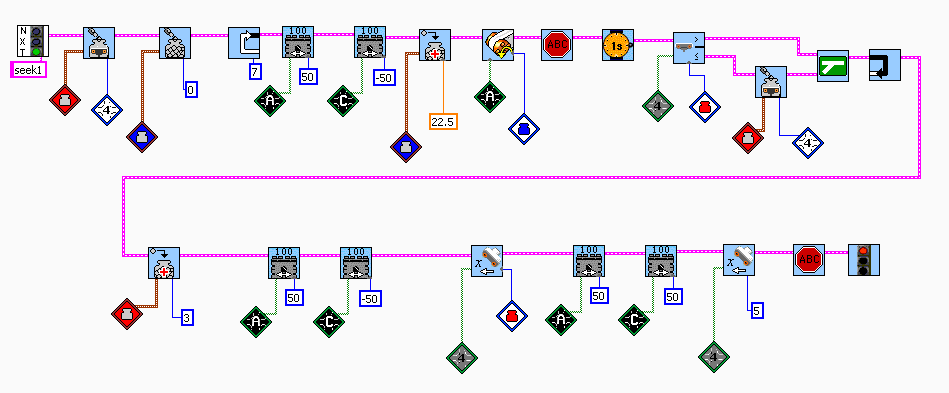Question 11: Write a program to make the car find the closest  object and then go toward that object. Stop at 5 cm from object. (Remember to break the problen down into small objectives, so the first thing to do is make the robot rotate in a circle. Next is to sample the distance with the ultrasonic sensor ever 45 degrees (based on rotationg sensor of one of the motors). Then create a container to hold the value, etc.

 Problem 11 needs containers and math again. Basically we want the robot to rotate in a circle and sample the distance every 45 degrees.  Take a distance sample and save it in a container.  Start rotating and taking samples. At each sample do some math to see if the distance is less than the distance saved in the container. If the new distance is less than the last sample, save the distance in a container.  After the robot has done a complete circle, the container has the closest distance.   Add 5 cm to the container. Do the circle again and stop when the distance is less than the container value. Drive forward until the distance is less than 5 cm and you are done.Return to All Questions